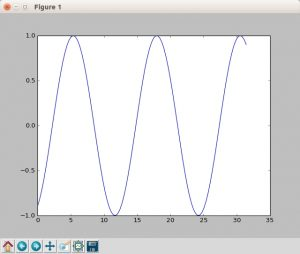# Matplotlib update plot

Python hosting: Host, run, and code Python in the cloud!

Updating a matplotlib plot is straightforward. Create the data, the plot and update in a loop.
Setting interactive mode on is essential: plt.ion(). This controls if the figure is redrawn every draw() command. If it is False (the default), then the figure does not update itself.

Related course:

## Update plot example

Copy the code below to test an interactive plot.Capture of a frame of the program above

Explanation
We create the data to plot using:

Turn on interacive mode using:

Configure the plot (the ‘b-‘ indicates a blue line):

And finally update in a loop: